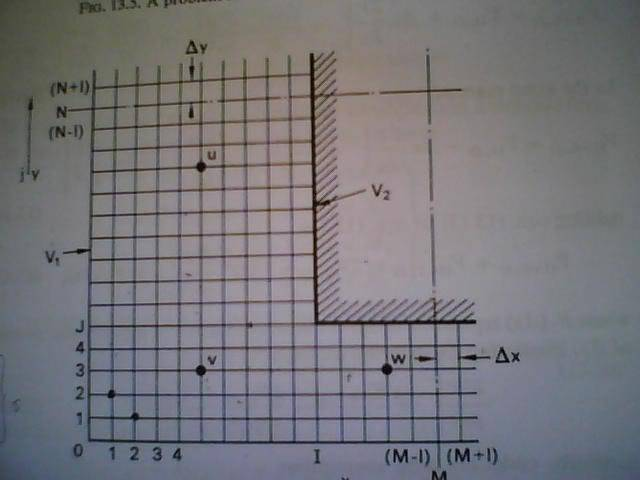# Finite difference method with matlab- square grid, cavity inside

• MATLAB
Hi,

I'm here for help and hope somebody could give a hand on this because I'm noob in this.

I'm now constructing a MATLAB program to find Electrical field and potential within a square grid mesh with square cavity inside.like the picture above.

I only manage up to this

v2=100.0;
%set no. of iteration
ni=1;
%nx=no. of x grid points
nx=8;
%ny=no. of y grid points
ny=6;
%set mesh size
d=5;
%nm=no of x grid for cavity
nm=4;
%nn=no of y grid for cavity
nn=3;
%set initial values equal to zeroes
v=zeros(nx+2,ny+2);
u=(nx-(nm-1));
t=(ny-(nm-1));

%fix potential at fixed nodes
for i=u:nx+1
v(i,t-1)=v2;
end
for j=t:ny+1
v(u-1,j)=v2;
end

head=['Iteration Coordinate Potential East North West South Electric field '
' Ex Ey'];

for k=1:ni
for u=2:nx+1
for t=2:ny+1
v(u,t)=0.25*(v(u,t)+v(u-1,t)+v(u,t+1)+v(u,t-1)+2*d^2);
Ex=(v(u+1,t)-v(u-1,t))/(2*d);
Ey=(v(i,u+1)-v(u,t-1))/(2*d);
fprintf('\t%5g \t(%g,%g) \t%5g \t%5g \t%5g \t%5g \t%5g \t%5g \t%5g \n ',k,u,t,v(u,t),v(u+1,t),v(u,t+1),v(u-1,t),v(u,t-1),Ex,Ey);
end
end
end

It seems that I'm now stuck where should i put a restriction to where the cavity is(that means, the program should not be calculating the V at that nodes)

can you give me some ideas how should i proceed with this?